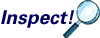# 9.2.3 - Optimal ClassificationPrinter-friendly version

For the moment, we will assume that we already have the covariance matrix for every class. And we will talk about how to estimate this in a moment.  Let's look at what the optimal classification would be based on the Bayes rule

Bayes rule says that we should pick a class that has the maximum posterior probability given the feature vector X. If we are using the generative modeling approach this is equivalent to maximizing the product of the prior and the within class density.

Since the log function is an increasing function, the maximization is equivalent because whatever gives you the maximum should also give you a maximum under a log function. Next, we plug in the density of the Gaussian distribution assuming common covariance and then multiplying the prior probabilities.

\begin{align*} \hat{G}(x) & = \text{arg } \underset{k}{\text{max}} Pr(G=k|X=x) \\ & = \text{arg } \underset{k}{\text{max}}f_k(x)\pi_k \\ & = \text{arg } \underset{k}{\text{max }} \text{ log}(f_k(x)\pi_k) \\ & = \text{arg } \underset{k}{\text{max}}\left[-\text{log}((2\pi)^{p/2}|\Sigma|^{1/2})-\frac{1}{2}(x-\mu_k)^T\Sigma^{-1}(x-\mu_k)+\text{log}(\pi_k) \right] \\ & = \text{arg } \underset{k}{\text{max}}\left[-\frac{1}{2}(x-\mu_k)^T\Sigma^{-1}(x-\mu_k)+\text{log}(\pi_k) \right] \end{align*}Note:

$-\frac{1}{2}(x-\mu_k)^T\Sigma^{-1}(x-\mu_k)=x^T\Sigma^{-1}\mu_k-\frac{1}{2}\mu_{k}^{T}\Sigma^{-1}\mu_k-\frac{1}{2}x^T\Sigma^{-1}x$

To sum up, after simplification we obtain this formula:

$\hat{G}(x)= \text{ arg }\underset{k}{max}\left[x^T\Sigma^{-1}\mu_k-\frac{1}{2}\mu_{k}^{T}\Sigma^{-1}\mu_{k} + log(\pi_k) \right]$

This is the final classifier. Given any x, you simply plug into this formula and see which k maximizes this.  Usually the number of classes is pretty small, and very often only two classes.  Hence, an exhaustive search over the classes is effective.

LDA gives you a linear boundary because the quadratic term is dropped.

To sum up

$\hat{G}(x)= \text{ arg }\underset{k}{max}\left[x^T\Sigma^{-1}\mu_k-\frac{1}{2}\mu_{k}^{T}\Sigma^{-1}\mu_{k} + log(\pi_k) \right]$

• Define the linear discriminant function

$\delta_k(x)=x^T\Sigma^{-1}\mu_k-\frac{1}{2}\mu_{k}^{T}\Sigma^{-1}\mu_{k} + log(\pi_k)$

$\hat{G}(x)= \text{ arg }\underset{k}{max}\delta_k(x)$

• Then

• The decision boundary between class k and l is:

$\left\{ x : \delta_k(x) = \delta_l(x)\right\}$

• Or equivalently the following holds

$log\frac{\pi_k}{\pi_l}-\frac{1}{2}(\mu_k+\mu_l)^T\Sigma^{-1}(\mu_k-\mu_l)+x^T\Sigma^{-1}(\mu_k-\mu_l)=0$